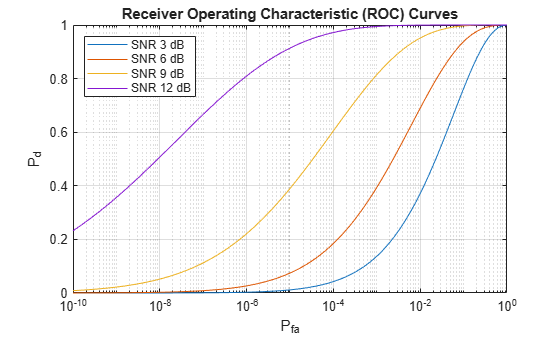# rocsnr

Receiver operating characteristic curves by SNR

## Syntax

```[Pd,Pfa] = rocsnr(SNRdB) [Pd,Pfa] = rocsnr(SNRdB,Name,Value) rocsnr(...) ```

## Description

```[Pd,Pfa] = rocsnr(SNRdB)``` returns the single-pulse detection probabilities, `Pd`, and false-alarm probabilities, `Pfa`, for the SNRs in the vector `SNRdB`. By default, for each SNR, the detection probabilities are computed for 101 false-alarm probabilities between 1e–10 and 1. The false-alarm probabilities are logarithmically equally spaced. The ROC curve is constructed assuming a coherent receiver with a nonfluctuating target.

```[Pd,Pfa] = rocsnr(SNRdB,Name,Value)``` returns detection probabilities and false-alarm probabilities with additional options specified by one or more `Name,Value` pair arguments.

`rocsnr(...)` plots the ROC curves.

## Input Arguments

 `SNRdB` Signal-to-noise ratios in decibels, in a row or column vector.

### Name-Value Arguments

Specify optional comma-separated pairs of `Name,Value` arguments. `Name` is the argument name and `Value` is the corresponding value. `Name` must appear inside quotes. You can specify several name and value pair arguments in any order as `Name1,Value1,...,NameN,ValueN`.

 `MaxPfa` Maximum false-alarm probability to include in the ROC calculation. Default: `1` `MinPfa` Minimum false-alarm probability to include in the ROC calculation. Default: `1e-10` `NumPoints` Number of false-alarm probabilities to use when calculating the ROC curves. The actual probability values are logarithmically equally spaced between `MinPfa` and `MaxPfa`. Default: `101` `NumPulses` Number of pulses to integrate when calculating the ROC curves. A value of `1` indicates no pulse integration. Default: `1` `SignalType` This property specifies the type of received signal or, equivalently, the probability density functions (PDF) used to compute the ROC. Valid values are: `'Real'`, `'NonfluctuatingCoherent'`, `'NonfluctuatingNoncoherent'`, `'Swerling1'`, `'Swerling2'`, `'Swerling3'`, and `'Swerling4'`. Values are not case sensitive. The `'NonfluctuatingCoherent'` signal type assumes that the noise in the received signal is a complex-valued, Gaussian random variable. This variable has independent zero-mean real and imaginary parts each with variance σ2/2 under the null hypothesis. In the case of a single pulse in a coherent receiver with complex white Gaussian noise, the probability of detection, PD, for a given false-alarm probability, PFA is: `${P}_{D}=\frac{1}{2}\text{erfc}\left({\text{erfc}}^{-1}\left(2{P}_{FA}\right)-\sqrt{\chi }\right)$`where `erfc` and `erfc-1` are the complementary error function and that function’s inverse, and χ is the SNR not expressed in decibels. For details about the other supported signal types, see . Default: `'NonfluctuatingCoherent'`

## Output Arguments

 `Pd` Detection probabilities corresponding to the false-alarm probabilities. For each SNR in `SNRdB`, `Pd` contains one column of detection probabilities. `Pfa` False-alarm probabilities in a column vector. By default, the false-alarm probabilities are 101 logarithmically equally spaced values between 1e–10 and 1. To change the range of probabilities, use the optional `MinPfa` or `MaxPfa` input argument. To change the number of probabilities, use the optional `NumPoints` input argument.

## Examples

collapse all

Plot ROC curves for different SNR's for a single pulse.

```SNRdB = [3 6 9 12]; [Pd,Pfa] = rocsnr(SNRdB,'SignalType','NonfluctuatingCoherent'); semilogx(Pfa,Pd) grid on xlabel('P_{fa}') ylabel('P_d') legend('SNR 3 dB','SNR 6 dB','SNR 9 dB','SNR 12 dB', 'location','northwest') title('Receiver Operating Characteristic (ROC) Curves')```## References

 Richards, M. A. Fundamentals of Radar Signal Processing. New York: McGraw-Hill, 2005, pp 298–336.